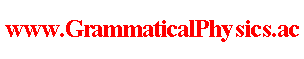since 2006 Help　Sitemap < Forum > < Problems > < Quantum History Theory > < Discretized Quantum Mechanical Solution > In this article, I treat the discrete time approximation of the new grammar version of Schrödinger equation in the case that V = 0. To do so, I use a sequence of numbers: ・・・, χ(-2/α), χ(-1/α), χ(0), χ(1/α), χ(2/α), ・・・ instead of a function χ representing a classical history. Accordingly, I replace the functional Φ in the equation with a function as follows. Φ[χ] → Φ(・・・, χ-2, χ-1, χ0, χ1, χ2, ・・・) where χk ≡χ(k/α). Moreover, I rewrite the original equation in the form of an equation for the following function F. Φ(χ) = F(a1(χ), b1(χ); a2(χ), b2(χ); a3(χ), b3(χ); ・・・) where an(χ) = Σk=-∞∞χk cos(kπ/n), bn(χ) = Σk=-∞∞χk sin(kπ/n). --- I calculate some differentiations as a preparation for a while. (d/dε)an(χ(□ - ε))|ε=0 ≒ α[an(χ(□ - 1/α)) - an(χ)] 　= αΣk=-∞∞(χk-1 - χk)cos(kπ/n) 　= αΣk=-∞∞χk[cos((k+1)π/n) - cos(kπ/n)] 　= αΣk=-∞∞χk[cos(kπ/n)cos(π/n) - sin(kπ/n)sin(π/n) - cos(kπ/n)] 　= α[cos(π/n) - 1]Σk=-∞∞χk cos(kπ/n) - αsin(π/n)Σk=-∞∞χk sin(kπ/n) 　= α[cos(π/n) - 1]an(χ) - αsin(π/n)bn(χ) (d/dε) bn(χ(□ - ε))|ε=0 ≒ α[bn(χ(□ - 1/α)) - bn(χ)] 　= αΣk=-∞∞(χk-1 - χk)sin(kπ/n) 　= αΣk=-∞∞χk[sin((k+1)π/n) - sin(kπ/n)] 　= αΣk=-∞∞χk[sin(kπ/n)cos(π/n) + cos(kπ/n)sin(π/n) - sin(kπ/n)] 　= α[cos(π/n) - 1]Σk=-∞∞χk sin(kπ/n) + αsin(π/n)Σk=-∞∞χk cos(kπ/n) 　= α[cos(π/n) - 1]bn(χ) + αsin(π/n)an(χ) (d/dε) Φ[χ(□ - ε)]|ε=0 　= Σn=1∞ [(dan/dε)∂F/∂an + (dbn/dε)∂F/∂bn]|ε=0 　= αΣn=1∞ [{[cos(π/n) - 1]an - sin(π/n)bn}∂F/∂an + {[cos(π/n) - 1]bn + sin(π/n)an}∂F/∂bn] ・・・ (1) This is a part of the left hand side of the original equation. --- To rewrite the right-hand side of the original equation, I do some calculations below as a preparation. ∂/∂χk = Σn=1∞[(∂an/∂χk)∂/∂an + (∂bn/∂χk)∂/∂bn} 　= Σn=1∞[cos(kπ/n)∂/∂an + sin(kπ/n)∂/∂bn] Σk=-∞∞(∂/∂χk)2 = Σk=-∞∞Σn=1∞Σs=1∞[cos(kπ/n)∂/∂an + sin(kπ/n)∂/∂bn][cos(kπ/s)∂/∂as + sin(kπ/s)∂/∂bs] 　= Σn=1∞Σs=1∞Σk=-∞∞[cos(kπ/n)cos(kπ/s)(∂/∂an)(∂/∂as) + sin(kπ/n)sin(kπ/s)(∂/∂bn)(∂/∂bs) + cos(kπ/n)sin(kπ/s)(∂/∂an)(∂/∂bs) + sin(kπ/n)cos(kπ/s)(∂/∂bn)(∂/∂as)] 　= (1/2)Σn=1∞Σs=1∞Σk=-∞∞[cos(kπ(1/n + 1/s)) + cos(kπ(1/n - 1/s))](∂/∂an)(∂/∂as) 　　+ (1/2)Σn=1∞Σs=1∞Σk=-∞∞[-cos(kπ(1/n + 1/s)) + cos(kπ(1/n - 1/s))](∂/∂bn)(∂/∂bs) 　　+ (1/2)Σn=1∞Σs=1∞Σk=-∞∞[sin(kπ(1/n + 1/s)) - sin(kπ(1/n - 1/s))](∂/∂an)(∂/∂bs) 　　+ (1/2)Σn=1∞Σs=1∞Σk=-∞∞[sin(kπ(1/n + 1/s)) + sin(kπ(1/n - 1/s))](∂/∂bn)(∂/∂as) Σk=-NN cos(kπ(1/n + 1/s)) = (Σk=-N-1 + Σk=00 + Σk=1N)cos(kπ(1/n + 1/s)) 　= Σk=1N cos(-kπ(1/n + 1/s)) + 1 + Σk=1N cos(kπ(1/n + 1/s)) 　= (1/2)sin[-(N + 1/2)π(1/n + 1/s)]/sin[-π(1/n + 1/s)/2] - 1/2 　　　+ 1 　　　+ (1/2)sin[(N + 1/2)π(1/n + 1/s)]/sin[π(1/n + 1/s)/2] - 1/2 　= sin[(N + 1/2)π(1/n + 1/s)]/sin[π(1/n + 1/s)/2] 　→ 0 (N → ∞) Σk=-NN sin(kπ(1/n + 1/s)) = (Σk=-N-1 + Σk=00 + Σk=1N)sin(kπ(1/n + 1/s)) 　= Σk=1Nsin(-kπ(1/n + 1/s)) + 0 + Σk=1Nsin(kπ(1/n + 1/s)) 　= 0 Σk=-NN cos(kπ(1/n - 1/s)) = (Σk=-N-1 + Σk=00 + Σk=1N)cos(kπ(1/n - 1/s)) 　= Σk=1N cos(-kπ(1/n - 1/s)) + 1 + Σk=1N cos(kπ(1/n - 1/s)) 　= (1/2)sin[-(N + 1/2)π(1/n - 1/s)]/sin[-π(1/n - 1/s)/2] - 1/2 　　　+ 1 　　　+ (1/2)sin[(N + 1/2)π(1/n - 1/s)]/sin[π(1/n - 1/s)/2] - 1/2 　= sin[(N + 1/2)π(1/n - 1/s)]/sin[π(1/n - 1/s)/2] 　→ 0 (N → ∞, n≠s) Σk=-NN cos(kπ(1/n - 1/s)) = (Σk=-N-1 + Σk=00 + Σk=1N)cos(kπ(1/n - 1/s)) 　= 2N + 1 (n = s) Σk=-NN sin(kπ(1/n - 1/s)) = (Σk=-N-1 + Σk=00 + Σk=1N)sin(kπ(1/n - 1/s)) 　= Σk=1Nsin(-kπ(1/n - 1/s)) + 0 + Σk=1Nsin(kπ(1/n - 1/s)) 　= 0 ∴ Σk=-∞∞(∂/∂χk)2 = limN→∞ [(2N + 1)/2]Σn=1∞[(∂/∂an)2 + (∂/∂bn)2] ・・・ (2) This is a part of the right hand side of the original equation. --- The original equation is rewritten as follows. (ih/α)(d/dε)Φ[χ(□ - ε)]|ε=0 = [1/(2m)](1/α)Σk=-∞∞[(-ih/α)α∂/∂χk]2Φ[χ] (d/dε)Φ[χ(□ - ε)]|ε=0 = [ih/(2m)]Σk=-∞∞(∂/∂χk)2Φ[χ] ・・・ (3) --- Substituting (1) and (2) into (3), the following equation is obtained. αΣn=1∞ [{[cos(π/n) - 1]an - sin(π/n)bn}∂F/∂an + {[cos(π/n) - 1]bn + sin(π/n)an}∂F/∂bn] = [ih/(2m)][(2N + 1)/2]Σn=1∞[(∂/∂an)2 + (∂/∂bn)2]F ∴ Σn=1∞{(∂/∂an)2 + (∂/∂bn)2 　　- {4mα/[(ih)(2N + 1)]}[{[cos(π/n) - 1]an - sin(π/n)bn}∂/∂an + {[cos(π/n) - 1]bn + sin(π/n)an}∂/∂bn]}F = 0 ・・・ (4) --- Here I specialize my investigation as F(a1, b1; a2, b2; a3, b3; ・・・) = f(an, bn). Then, (4) reduces as follows. {(∂/∂ξ)2 + (∂/∂η)2 - [(βξ - γη)∂/∂ξ + (βη + γξ)∂/∂η]}f(ξ,η) = 0 ・・・ (5) where β = {4mα/[(ih)(2N + 1)]}[cos(π/n) - 1], γ = {4mα/[(ih)(2N + 1)]}sin(π/n),N is a number to tend to infinity. --- To solve Equation (5), I rewrite it in the form of an equation for the following function g. f(ξ,η) = ∫dp∫dq g(p, q)exp(ipξ + iqη). ξ(∂/∂ξ)f(ξ,η) = ∫dp∫dq g(p, q)ipξexp(ipξ + iqη) = ∫dp∫dq g(p, q)ip(-i)(∂/∂p)exp(ipξ + iqη) = -∫dp∫dq exp(ipξ + iqη)(∂/∂p)[p g(p, q)] η(∂/∂ξ)f(ξ,η) = ∫dp∫dq g(p, q)ipηexp(ipξ + iqη) = ∫dp∫dq g(p, q)ip(-i)(∂/∂q)exp(ipξ + iqη) = -∫dp∫dq exp(ipξ + iqη)(∂/∂q)[p g(p, q)] η(∂/∂η)f(ξ,η) = ∫dp∫dq g(p, q)iqηexp(ipξ + iqη) = ∫dp∫dq g(p, q)iq(-i)(∂/∂q)exp(ipξ + iqη) = -∫dp∫dq exp(ipξ + iqη)(∂/∂q)[q g(p, q)] ξ(∂/∂η)f(ξ,η) = ∫dp∫dq g(p, q)iqξexp(ipξ + iqη) = ∫dp∫dq g(p, q)iq(-i)(∂/∂p)exp(ipξ + iqη) = -∫dp∫dq exp(ipξ + iqη)(∂/∂p)[q g(p, q)] The equation ia rewritten as follows. [-p2 -q2 + (β∂/∂p - γ∂/∂q)p + (β∂/∂q + γ∂/∂p)q]g(p, q) = 0. ∴ [-p2 -q2 + p(β∂/∂p - γ∂/∂q) + q(β∂/∂q + γ∂/∂p) +2β]g(p, q) = 0 ・・・ (6) --- To solve Equation (6), I rewrite it in the form of an equation for the following function h. g(p, q) = h(r, θ) where p = r cosθ, q = r sinθ. ∂/∂r = (cosθ)∂/∂p + (sinθ)∂/∂q, ∂/∂θ = -r(sinθ)∂/∂p + r(cosθ)∂/∂q ∂/∂p = (cosθ)∂/∂r - (1/r)(sinθ)∂/∂θ, ∂/∂q = (sinθ)∂/∂r + (1/r)(cosθ)∂/∂θ p∂/∂p + q∂/∂q = r∂/∂r, q∂/∂p - p∂/∂q = -∂/∂θ The equation ia rewritten as follows. (-r2 + βr∂/∂r - γ∂/∂θ + 2β)h(r, θ) = 0 ・・・ (7) --- To solve Equation (7), I rewrite it in the form of an equation for the following function R. h(r, θ) = Σk=-∞∞ Rk(r)exp(ikθ). The equation is rewritten as follows. (-r2 + βr∂/∂r - iγk + 2β)Rk(r) = 0 ・・・ (8). The solution of this equation is as follows. Rk(r) = λk[r^(-2 + iγk/β)]exp[r2/(2β)] ・・・ (9). Author Yuichi Uda, Write start at 2015/10/03/01:48JST, Last edit at 2016/02/07/19:52JST